Courses

# Linear Algebra NAT Level - 1

## 10 Questions MCQ Test Topic wise Tests for IIT JAM Physics | Linear Algebra NAT Level - 1

Description
This mock test of Linear Algebra NAT Level - 1 for IIT JAM helps you for every IIT JAM entrance exam. This contains 10 Multiple Choice Questions for IIT JAM Linear Algebra NAT Level - 1 (mcq) to study with solutions a complete question bank. The solved questions answers in this Linear Algebra NAT Level - 1 quiz give you a good mix of easy questions and tough questions. IIT JAM students definitely take this Linear Algebra NAT Level - 1 exercise for a better result in the exam. You can find other Linear Algebra NAT Level - 1 extra questions, long questions & short questions for IIT JAM on EduRev as well by searching above.
*Answer can only contain numeric values
QUESTION: 1

### What is the maximum value of λ, for which the given system is consistent?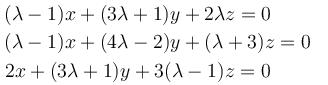Solution:

The given system will be consistent if,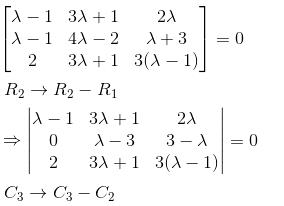*Answer can only contain numeric values
QUESTION: 2

### Given a matrix,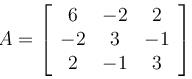What would be the sum of eigenvalues of A–1?

Solution: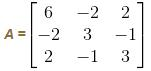The characteristic equation of A is,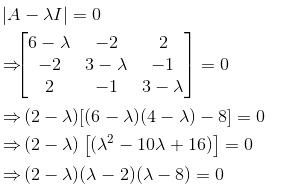∴   the characteristic root of A are given by

λ = 2, 2, 8

We know that if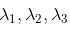are the eigenvalues of  A, then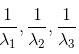will be the eigenvalues of A–1.

Hence, in this case, eigenvalues of  A–1 will be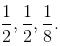∴  Sum of eigenvalues of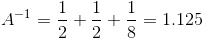*Answer can only contain numeric values
QUESTION: 3

### Consider the matrix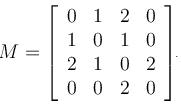Then find the product of eigenvalues?

Solution: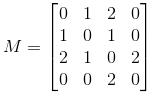The eigenvalues of M are given by :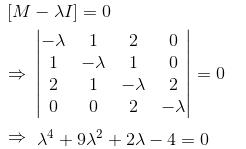⇒ Product of eigenvalues = 4
So, M  both positive and negative real eigenvalues.

*Answer can only contain numeric values
QUESTION: 4

The three equations,
–2x + y + z = a
x – 2y + z = b
x + y – 2z = c
has no solution. Find the value of  (a + b + c)

Solution: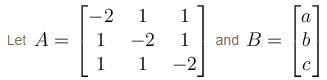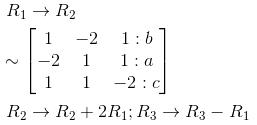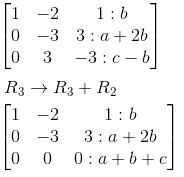Hence, the system won't contain any solution unless a + b + c becomes 0.

*Answer can only contain numeric values
QUESTION: 5

Consider the following equations
x + y – 3z + 2w = 0
2x – y + 2z – 3w = 0
3x – 2y + z – 4w = 0
–4x – y + 3z + 4w = 0

Find the rank of the coefficient matrix.

Solution:

Consider the coefficient matrix, A of the given system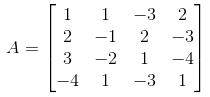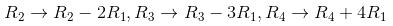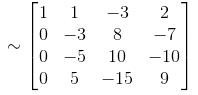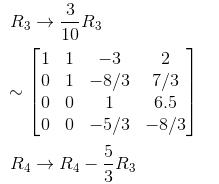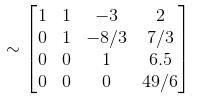Hence, rank of  A = 4 = Number of unknowns

*Answer can only contain numeric values
QUESTION: 6

If x = y = z = αc satisfy the matrix equation AX = 0 where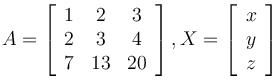then find the value of α.

Solution: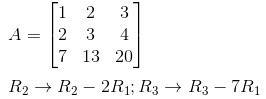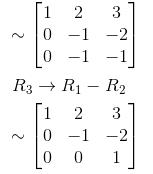⇒  Rank of A = 2 and z  is a free variable. The given system reduces to
x + 2y + 3z = 0
y – 2z = 0
Let  z = 0
⇒ y = 0
and  x = –2y – 3z = 0
Hence,  x = y = z = 0  gives the general solution.
⇒  α = 0

*Answer can only contain numeric values
QUESTION: 7

For which value of λ will the following equations fail to have unique solution?

3x – y + λz = 1
2x + y + z = 2
x + 2y – λz = –1

Solution:

The matrix form of the given system of equations is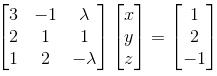The given system of equations will have a unique solution if and only if the coefficient matrix is non singular.

Performing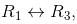we get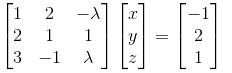Performing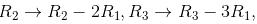we get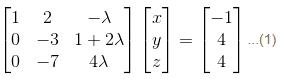Therefore, the coefficient matrix will be non-singular if and only if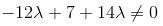i.e., if and only if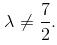Thus, the given system will have a unique solution if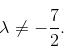In case of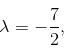the equation (1) becomes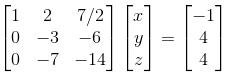Performing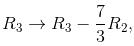we get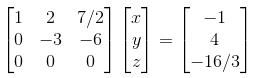showing that given equations are inconsistent in this case.

Thus if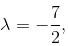no solution exists.

*Answer can only contain numeric values
QUESTION: 8

Let A be a 3 × 3 matrix with eigen values 1, –1, 0. Then the determinant of I + A100 is :

Solution:

Eigen values of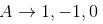⇒ Eigen values of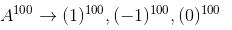Eigen values of = 1, 1, 0
Eigen values of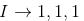⇒  Eigen values of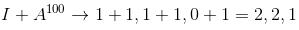Hence,  |A100 + I| = 2 × 2 × 1 = 4

*Answer can only contain numeric values
QUESTION: 9

The system of equations αx + y + z = α - 1, x + αy + z = α - 1, x + y + αz = α - 1 has no solution. Find the maximum value of α

Solution:

The given system is,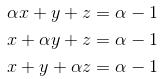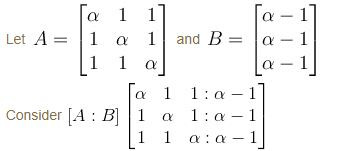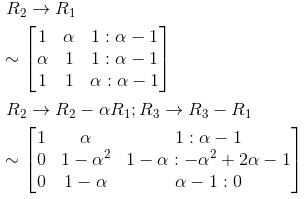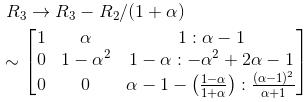The system won't have any solution if rank (A) ≠ Rank(A : B) i.e.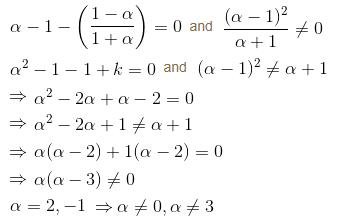∴   α = either 2 or –1 but not equals to 0 or 3.

*Answer can only contain numeric values
QUESTION: 10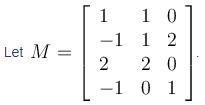Then the rank of M is equal to :

Solution:

The given matrix is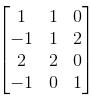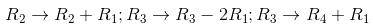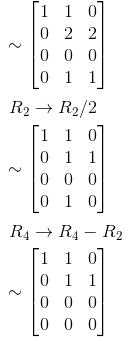Hence rank M = 2